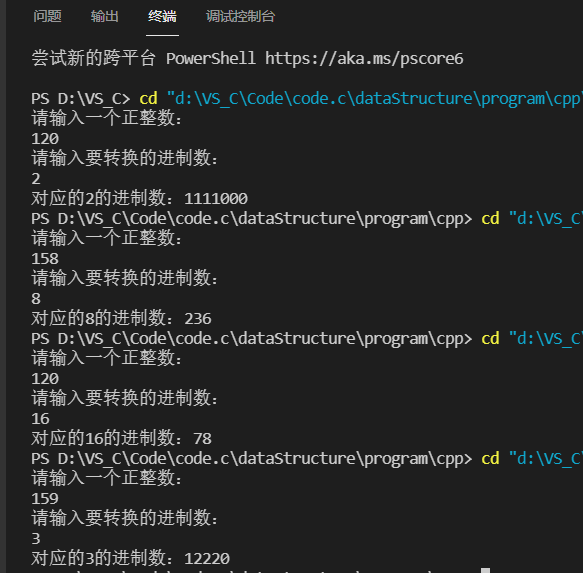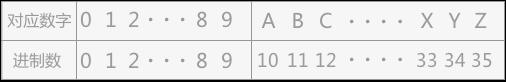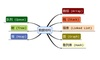• 2021-10-31 21:04:33

思路：

将输入的数据,先转换为10进制数,再转换成期望的数

#include<stdio.h>
#include<math.h>
#include<string.h>
#include<stdlib.h>

typedef struct Node {
char *base;//指向栈底
char *top;//指向栈顶
int size;
//size = 99;
}*stack;

stack init(){//栈的初始化
//int n;
stack s = (stack)malloc(sizeof(stack));
s->base = (char*)malloc(sizeof(char)*64);
if(!s->base)
return NULL;
//scanf("%d",&n);
s->size = 64;
printf("初始化成功\n");
s->top = s->base;
s->size = 64;
return s;
}

int full_stack(stack s){//判断是否栈空
if(s->top - s->base == 64)
return 1;
else
return 0;
}

int empty_stack(stack s){
if(s->top == s->base)
return 1;
else
return 0;
}

void push_stack(stack s,char n){//压栈

if(full_stack(s) == 1){
printf("已经栈满,无法添加!\n");
return;
}
else {
*(s->top++) = n;
//	printf("入栈成功\n");
}
}

void pop_stack(stack s,char*e){
if(empty_stack(s) == 1){
printf("已经栈空!\n");
return ;
}
else{
*e = *(--s->top);
}
}

char* BinChange(stack s,int n);
char* OctChange(stack s,int n);
char* HexChange(stack s,int n);
char* Change(stack s,int n,int q);
int DecChange(stack s,char*p,int q);

void judge(){
stack s = init();
printf("请输入待转换数据的类型:(2,8,16)\n");
int q,n;
scanf("%d",&q);
printf("请输入您要转换的数据:\n");
char arr;
scanf("%s",arr);
char*temp = arr;
int i;
if(q<16)
for(i=0;i<strlen(arr);i++){
if((int)arr[i]-48>= q || (int)arr[i]-48< 0)
{
printf("%d\n",(int)arr[i]-48);
printf("%d\n",i);
printf("数据类型不匹配!");
return;
}
}else
for(i=0;i<strlen(arr);i++)
if(	(	(int)arr[i]-48>=9 && ((int)arr[i]-55<=0 ||(int)arr[i]-55>=q) ) || (int)arr[i]-48<=0)
{
printf("数据类型不匹配!");
return;
}
char*p = (char*)malloc(sizeof(char)*64);
char*p1 = (char*)malloc(sizeof(char)*64);
strcpy(p,arr);
printf("请输入期望的类型:\n");
scanf("%d",&n);
int tot;
tot = DecChange(s,p,q);
printf("十进制后为%d\n",tot);
stack sp = init();
switch(n){
case 2: p1 = BinChange(sp,tot);break;
case 8: p1 = OctChange(sp,tot);break;
case 16: p1 = HexChange(sp,tot);break;
default : p1 = Change(sp,tot,n);break;   //printf("输入非法!\n")
}
}

int main(){
judge();
}

char* BinChange(stack s,int n)//转二进制
{
int temp;
int i,cnt;
char ch = '0';
char *p = malloc(sizeof(char)*64);
while(n!=0){
temp = n/2;
i = n-temp*2;
printf("%c",(char)ch+i);
push_stack(s,ch+i);
cnt++;
n/=2;
}
while(empty_stack(s)!=1){
//printf("!!!\n");
pop_stack(s,&ch);
*p++ = ch;
printf("%c",*(p-1));
}
return p;
}
char* OctChange(stack s,int n){
int temp;
int i,cnt;
char ch = '0';
char *p = malloc(sizeof(char)*64);
while(n!=0){
temp = n/8;
i = n-temp*8;
push_stack(s,ch+i);
cnt++;
n/=8;
}
while(empty_stack(s)!=1){
pop_stack(s,&ch);
*p++ = ch;
printf("%c",*(p-1));
}
return p;
}
char* HexChange(stack s,int n){
int temp;
int i,cnt;
char ch = '0';
char *p = malloc(sizeof(char)*64);
while(n!=0){
temp = n/16;
i = n-temp*16;
if(i<10 && i>0)
push_stack(s,ch+i);
if(i>=10 && i<15 )
{
i-=10;
push_stack(s,'A'+i);
}
cnt++;
n/=16;
}
while(empty_stack(s)!=1){
pop_stack(s,&ch);
*p++ = ch;
printf("%c",*(p-1));
}
return p;
}

char* Change(stack s,int n,int q){
int temp;
int i,cnt;
char ch = '0';
char *p = malloc(sizeof(char)*64);
while(n!=0){
temp = n/q;
i = n-temp*q;//判断除以q后的余数
if(i<10 && i>0)
push_stack(s,ch+i);
if(i>=10 && i<q-1 )
{
i-=10;
push_stack(s,'A'+i);
}
cnt++;
n/=q;
}
while(empty_stack(s)!=1){
pop_stack(s,&ch);
*p++ = ch;
printf("%c",*(p-1));
}
return p;
}

int DecChange(stack s,char*p,int q){
int len = strlen(p);
int i;
char temp='0';
int cap=0;
for(i=0;i<len;i++){

//printf("\np ASCII:%d\n",(int)*p-48);
push_stack(s,*p++);
//printf("%c\n",*(s->top-1));
}
for(i=0;i<len;i++){
if(q!=16){
pop_stack(s,&temp);
//printf("temp:%c\n",temp);
cap+=((int)pow(q,i)*((int)temp-48));
//printf("cap:%d\n",cap);

}
if(q==16){
pop_stack(s,&temp);
if((int)temp-48<=9 && (int)temp-48>=0)
{

//printf("temp<10:%c\n",temp);
cap+=((int)pow(q,i)*((int)temp-48));
//printf("cap:%d\n",cap);

}
else if((int)temp-55>=0 && (int)temp-55<=q)
{

//printf("temp>10:%c\n",temp);
cap+=((int)pow(q,i)*((int)temp-55));
//printf("cap:%d\n",cap);
}
}

}
return cap;
}c语言
更多相关内容
• 进制转换一直是保研机试的时候的热门考点，本文来介绍进制转换的处理方法。

# 1.  目标

进制数转换一直是保研机试的时候的热门考点，本文来介绍进制数转换的处理方法。

# 2. 简单情况

我们首先来考虑最简单的十进制数转换二进制数的情况。

## 2.1  问题分析

结合数学知识，我们知道在进制转换的过程结束后，要把过程中得到的结果逆序输出。考虑具有这种特性的数据结构，我们自然可以想到使用栈的先入后出的特性来解决这个问题。当然，我们也可以使用向量甚至是数组来解决这个问题，此处为了方便，我们使用STL中的stack来解决。

## 2.2 代码

//十进制数转二进制数

#include<iostream>
#include<cstdio>
#include<stack>

using namespace std;

int main()
{
int n;
stack<int> arr;
while (scanf("%d", &n) != EOF)
{
int data, tmp;
tmp = n;
while (tmp != 0)
{
data = tmp % 2;
arr.push(data);
tmp /= 2;
}
printf("%d = ", n);
while (!arr.empty())
{
printf("%d", arr.top());
arr.pop();
}
printf("\n");
}
return 0;
}

# 3. 复杂情况

下面我们来考虑任意进制数之间的转换。

## 3.1 问题分析

我们计划将这个问题分为以下步骤来解决：

• 输入数据（字符） 十进制数（整型）
• 十进制数（整型） 输出数据（字符）

考虑到输入数据可能含有字母，所以我们事先使用string存储输入数据。同理，输出数据可能也含有字符。

## 3.2 问题实现

### 3.2.1 样例

输入：

输入的第一行包括两个整数：M和N（2<=M,N<=36）.
下面的一行输入一个数X，X是M进制的数，现在要求你将M进制的数X转换成N进制的数并输出。

输出：

输出X的N进制表示的数。

样例：

10 2
11
11 = 1011

### 3.2.2 代码

//任意进制数的转换

#include<iostream>
#include<cstdio>
#include<string>
#include<stack>

using namespace std;

char IntToChar(int x)
{
if (x < 10)
{
return x + '0';
}
else
{
return x - 10 + 'a';
}
}

int CharToInt(char c)
{
if (c >= '0'&&c <= '9')
{
return c - '0';
}
else if (c >= 'A' && c <= 'Z')
{
return c - 'A' + 10;
}
else if (c >= 'a' && c <= 'z')
{
return c - 'a' + 10;
}
else
{
printf("error");
exit(0);
}
}

int main()
{
int m, n;
scanf("%d%d", &m, &n);
string str;
cin >> str;
long long number = 0;
for (int i = 0; i < str.size(); i++)
{
number *= m;
number += CharToInt(str[i]);
}
while (number != 0)
{
number /= n;
}
cout << str << " = ";
{
}
printf("\n");

return 0;
}

展开全文c++
• 算法与数据结构实验报告 实验三 实验名称: 栈和队列进制转换 姓名 X X X 学号 211106365 专业 软件工程 班级 X班 指导教师 X X X 日期: 2013年 月 日 实验目的 学会应用栈或队列解决进制转换问题熟练掌握在顺序栈...
• C++语言实现带小数的任意进制转换，使用了数据结构中的栈和队列，在VC++6.0上编译运行通过。对于学习C++和数据结构有一定的参考意义！
• 我的其他资源都是免费的，是对于c语言初学者的帮助比较大的，其中有单片机，ARM，数据结构，window编程，MFC编程，自己编写的小游戏。
• } //进制转换 void trans(int d, int c, char b[]) { SqStack S; InitStack(S); char ch; int i = 0; while (d != 0) { ch = '0' + d % c; //求余数并转为字符 Push(S, ch); //进栈 d /= c; //继续下一位 } while ...

先上结果图：代码部分：

/*
Author:LCH南安
Time：2021.10.25

*/
#include <stdio.h>
#include <stdlib.h>
#include <string.h>
#define STACK_INIT_SIZE 100

#define MaxSize 100;   //可用最大容量
typedef char ElemType; //定义为char类型
typedef struct SqStack
{
ElemType *base; //栈底指针
int top;        //栈顶
int stacksize;  //栈可用最大容量
} SqStack;
//构造一个空的栈
void InitStack(SqStack &S)
{ //分配空间大小为STACK_INIT_SIZE的栈
S.base = (ElemType *)malloc(STACK_INIT_SIZE * sizeof(ElemType));

if (!S.base)
exit(0); // 存储分配失败
S.top = -1;  //栈空

S.stacksize = MaxSize; //栈可用最大容量为100
}
//元素进栈
int Push(SqStack &S, ElemType e)
{
if (S.top >= S.stacksize - 1) //判断是否溢出
{
//S.base=(ElemType *)realloc(S.base,(S.stacksize+STACK_INIT_SIZE)*sizeof(ElemType));
//if(!S.base) exit();
//S.top=S.base+S.stacksize;                //由于realloc可能不在原malloc分配的起始地址开始，
//可能从另一足够大分区开始重新分配内存,所以必须更新top;
//S.stacksize+=STACK_INIT_SIZE

return 0;
}
S.top++;
S.base[S.top] = e; //进栈
return 1;
}
//元素出栈
int Pop(SqStack &S, ElemType &e)
{
if (S.top == -1) //判断是否栈空
{
return 0;
}
else
{
e = S.base[S.top]; //出栈，e接收
S.top--;           //栈顶降低

return 1;
}
}
//判断栈是否为空
int StackEmpty(SqStack S)
{
if (S.top == -1) //判断是否栈空
return 1;
else
return 0;
}
//销毁顺序栈
int DestroyStack(SqStack &S)
{
if (S.base)
{
delete S.base;
S.stacksize = 0; //最大容量变为0
S.top = -1;      //栈顶标志
S.base = NULL;   //栈底指针为NULL
}
return 1;
}
//进制转换
void trans(int d, int c, char b[])
{
SqStack S;
InitStack(S);
char ch;
int i = 0;
while (d != 0)
{
ch = '0' + d % c; //求余数并转为字符
Push(S, ch);      //进栈
d /= c;           //继续下一位
}
while (!StackEmpty(S)) //判栈空
{
Pop(S, ch); //出栈
b[i] = ch;  //将出栈元素数组
i++;
}
b[i] = '\0';     //加入字符串结束标志
DestroyStack(S); //销毁S
}

int main()
{

int d, c;
char str;

do
{
printf("请输入一个正整数：\n"); //保证输入正整数
scanf("%d", &d);
printf("请输入要转换的进制数：\n");
scanf("%d", &c);
} while (d < 0);
trans(d, c, str); //传参
printf("对应的%d的进制数：%s\n", c, str);
return 0;
}

展开全文c++ 开发语言
• 进制转换器项目要求：用户提供需要转换的数据和该数据的进制，以及要转换的进制，进制转换器提供给用户最终的正确转换的结果。 转换器实例 例如，用户提供了一个十进制数：10，要求将此数据以二进制形式转换，则...

进制转换器项目要求：用户提供需要转换的数据和该数据的进制，以及要转换的进制，进制转换器提供给用户最终的正确转换的结果。

转换器实例

例如，用户提供了一个十进制数：10，要求将此数据以二进制形式转换，则通过进制转换器转换的最终结果应该：1010。

提示：此进制转换器可以在

2-36 进制之间对数据进行任意转换。各进制中对应的数字如下表：设计思路
当用户给定 2 - 36 进制中的任意一进制数时，最简单的方法是使用顺序存储结构进行存储，即使用字符串数组存储。

转化时，最直接的思路就是先将该数转化为十进制数据，然后再由十进制转化成要求的进制数，最终的结果用栈结构存储（先进后出），这样最终显示
给用户的是正常的数据。

实现代码

#include <stdio.h>
#include <string.h>
#include <math.h>
int top=-1;//top 变量时刻表示栈顶元素所在位置
void push(char * a,char elem){
a[++top]=elem;
}
void pop(char * a){
if (top==-1) {
return ;
}
//输出时要按照正确的格式显示给用户
if (a[top
展开全文c++ c语言 算法 经验分享
• 进制转换二进制（代码已经给大家，详情参考“PPT-Jk17数据结构第二次上机任务-实验3-2018年9月26日”） 实现十进制与任意进制的转换(10以内) 用顺序表和链表两种数据结构实现上述两类功能 1.顺序栈实现十进制转化...
•散列表 leetcode
• 任意进制转换十进制 – 数据结构c语言队列操作实现 代码也不算是任意进制，最高只能到十六进制。见谅！ 代码如下： #include <stdio.h> #include <stdlib.h> #include <string.h> #define MAXSIZE...队列 c语言 十进制
• 源代码来的，经过运行唔错的，想交作业或者懒得去计算的可以下载去玩玩
• 采用链栈， 基本流程为：先将任意进制转化为十进制，再将十进制转化为目标进制， 达到任意进制转化任意进制的功能。 中间函数：将任意进制转化为十进制 int Anysystem_convert_TenSystem(char *s,int n) { int ...c++
• 整数转换任意进制 我们用最熟悉的十进制分析下这个问题 十进制有十个不同符号： convString ="0123456789"比十小的整数，转换成十进制， 直接查表就可以了： convString[n]想办法把比十大的整数，拆成一系列比十小...算法 python
• 1、将任意进制转换为三种进制数（二进制、八进制、十六进制）。 2、输入任意一串由括号（、）、[、]、{、}组成的括号序列，判断括号是否匹配。 文件包含源代码和实验报告，环境为vs2019，实验报告中含有实验目的...
• 进制有十个不同的符号：convString='0123456789'，比10小的数转换成十进制，直接查表就可以：convString[n]，那么比10大的整数该怎么解决呢？ 根据递归三定律，我们找到基本结束条件，就是小于10的整数，拆解整数...
• 我们都熟悉任意进制和十进制之间的转换任意进制转换的思路就是以十进制为桥梁，先把数字转成十进制，再把十进制转成目的进制。 任意进制转十进制，比如：45(8)，转成十进制，其 result = 4x81+5x80 = 32+5 = 37...
• 有趣的数据结构算法9——利用栈完成2进制到8进制转换解题思路实现代码GITHUB下载连接 刚学习完栈的我想试试栈都可以干嘛，于是找到了一个小应用，利用栈完成2进制到8进制转换。如果大家还不清楚什么是栈、如何...
• 前言：上学期的数据结构课设写的，拿了优秀（代价就是爆肝完拉了两天肚子），代码如下，功能十分齐全。其实只要明白进制转换的过程就能写出来，下面代码分别实现了 1.十进制数转换成任意进制数 2.任意进制数转换成十...程序设计 c语言 算法
• 所以，为了方便，我们在进制转换过程中，不过是几进制转换几进制，统统把原数据转换为十进制，然后再转换为目标进制。这样我们就可以通过两个函数就可以实现进制转换功能。 对于任意进制数转换为十进制数，只需要按...算法
• 本题需要将十进制整数num转换任意进制数，要求使用链栈实现转换。程序中设计了四个函数，(1)函数InitStack()用来初始化一个顺序栈，(2)函数Empty()用来实现栈的判空操作，(3)函数Pop()用来实现元素的出栈操作，(4)...c语言
•c++
• * 主题 ：对于输入的任意一个非负十进制小数，打印输出与其等值的任意进制小数 * Date : 2018/10/14 */ #include &lt;stdio.h&gt; #include &lt;stdlib.h&gt; #include &lt...
• 数据结构C++ 任意进制进制转换 源文件cpp 用链栈实现
• /*功能：将十进制转换成十六进制数。-------------------------------------------------------*/#include "stdio.h"#include "string.h"void main (){int a,i,n;char s;printf("input a integer:\n");scanf(...
• 符合进制转换的方式，故用栈这种结构。 一.定义声明 #include<iostream> using namespace std; #define OK 1 #define ERROR 0 typedef int Status; typedef int ElemType; //定义结构体： typedef struct ...c++
• 一、八进制的142转换成十进制 1*8^2 + 4*8 + 2*8^0 = 64 +...三、C++ Stack实现十进制到任意进制转换代码 #include <iostream> #include <stack> using namespace std; void convert(stack<char&...c++ stack类
• ## C语言实现任意进制间的转换

千次阅读 多人点赞 2020-02-05 12:07:20
C语言实现任意进制间的转换可以以十进制为基准，先将需要转换的数字转换成十进制然后再将其转换成目标进制数，这样就实现了任意进制间的转换。 引用函数： int ChangeTen(int n, char str[]); ChangeX(int n, int ...
• 先将a进制转换为10进制，再将该十进制数转换为b进制。 特别注意的是，超过10进制的数有用字母表示，所以栈应该存字符。 而且要转换的数据可能是超过10进制的数，所有用字符串表示。 #include <iostream> #...
• 进制转换用栈实现 #include<iostream> #include<stdio.h> #include<stdlib.h> #include<string.h> using namespace std; #define MAXSIZE 100 enum Status{ OK=1, ERROR=0, OVERFLOW=-1 ...c语言 c++ 算法 链表...

# 数据结构 任意进制转换数据结构 订阅